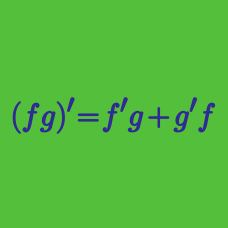Calculus

# Differentiation - Power Rule

What is the derivative of $\displaystyle y=5 x^{3}+\frac{1}{x^{6}}$?

What is the derivative of $-17 x^{16}$?

If $f(x) = \displaystyle{\sum_{k=1}^{n} x^k},$ what is $\displaystyle{ \lim_{n \rightarrow \infty} \frac{f'(1)}{3n^2+4n+5} }?$

What is the derivative of $y = \frac{4}{x}+\frac{4}{x^2}?$

What is the derivative of $x^{15}$?

×